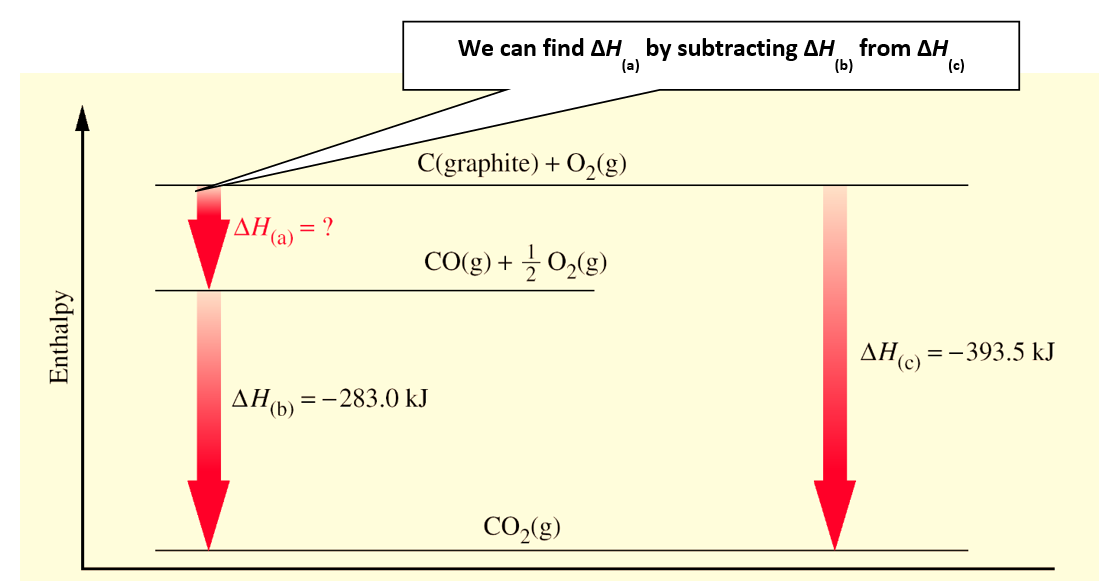# Hess’s Law of constant heat summation

Chemical Thermodynamics: Hess’s Law of constant heat summation

Hess’s Law of Constant Heat Summation states that the total enthalpy of a chemical reaction can be depicted as the sum of the enthalpy changes for the steps of the reaction. Therefore, you can find enthalpy change by breaking a reaction into component steps that have known enthalpy values.If an equation can be expressed as the sum of two or more other equations, the enthalpy change for the desired equation is the sum of the enthalpy changes of the other equations.Hess’s Law is acceptable and valid because ∆H is a state function.To apply Hess’s Law, all the steps of a chemical reaction should occur at the same temperature.Figure 1: Hess’s law of summation

Some reactions cannot be carried out “as written.”

Consider the reaction:

C(graphite) + ½ O2(g)  CO(g).

If we burned 1 mol C in ½ mol O2, both CO and CO2 would probably form. Some C might be left overenthalpy change is a state function. The enthalpy change of a reaction is the same whether the reaction is carried out in one step or through a few steps.We can find ∆H(a) by subtracting ∆H(b) from ∆H(c)

The direction of enthalpy can change depending on how the reaction is written and on phases of reactants and products. For example:H2(g) + 1/2 O2(g) → H2O(g) ∆H˚ = -242 kJ

2H2(g) + O2(g) → 2H2O(g) ∆H˚ = -484 kJ

or

H2O(g) → H2(g) + 1/2 O2(g) ∆H˚ = +242 kJ

H2(g) + 1/2 O2(g) → H2O(l) ∆H˚ = -286 kJ

Steps involved in solving enthalpy of combustion problems:

1. Balance the individual equations
2. If necessary, look up standard enthalpies
3. Flip equations around if necessary, to cancel out terms on opposite sides
4. Changing the equation around requires a sign change of the H of that individual step
5. Sum up the individual steps

Hess’s Law can also be used to determine other state functions such as gibbs free energy and entropy.

For example,the Bordwell thermodynamic cycle takes advantage of easily measured equilibriums and the redox potentials to find out the experimentally unattainable Gibbs free energy values.

G(reaction) = ∑n△G(product)–∑n△G(reactants)

As entropy can be measured as an absolute value, hence in case of entropy there is no need to use the entropy of formation.

S(reaction) = ∑nS(products)–∑nS(reactants)

In this equation, the symbol Σ, Greek letter sigma and means “the sum of”. This equation explains that the standard heat of reaction is equal to the sum of all the standard heats of formation of the products minus the sum of all the standard heats of formation of the reactants. The symbol “n” signifies that each heat of formation must first be multiplied by its coefficient in the balanced equation.

Applications of Hess’s Law:

• Thermochemical equations can be added subtracted or multiply like ordinary algebraic equations
• Hess’s law is useful to calculate heat involved in many difficult to measure reactions
• It is useful in the determination of heat of formation, reaction, transition, neutralization etc.
• It is useful in the determination of heats of extremely slow reaction